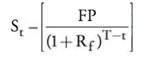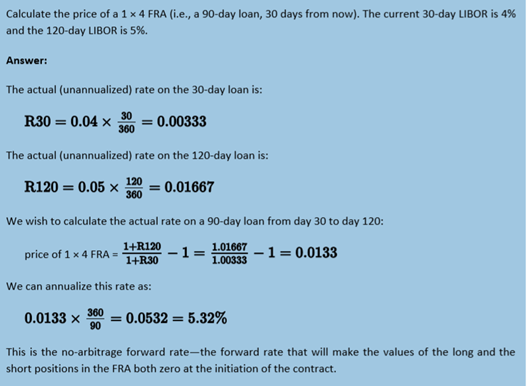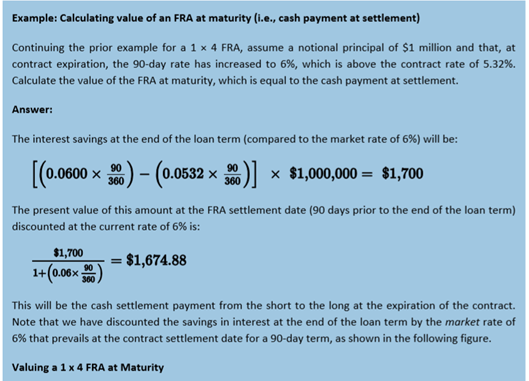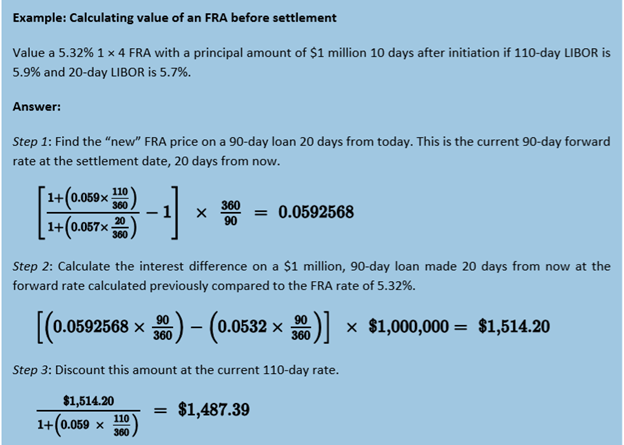# Module 39.5: Valuing FRAs

#### Price vs Value of Forward Contracts

First we look at the difference between the price of the forward contract and the value of a forward contract. The price of the contract is fixed. It represents the rate that the long and short side have agreed to transact the underlying asset for at expiration of the contract.

The value of forward contract is the amount of money that one side of the trade would be willing to pay the counterparty to exit the contract.

The no-arbitrage price of a forward contract is price where the value of the long side and the short side are equal to zero. At the initiation the value of contract is 0, which is done to prevent arbitrage.

• Forward Price = S0 x (1+Rf)T
• Where S0 is the spot price of underlying at inception

During the life of the contract the value of the long position can be denoted as

•The value of the long position at time t is the current underlying spot price minus the initial FP discounted by the risk-free rate to the time remaining on the contract.

At the expiration, the long position value of the contract = ST – FP.

Because forward contracts are zero sum games, the value of the short is just the negative value of the long.

#### Forward Rate Agreements

An FRA is an OTC contract between parties that determines the currency or interest rate that will paid or received on the obligation starting on a future date.

Per the material, the long side on an FRA is the party that wants to borrow money at the contract price, which would be the interest rate agreed on. If the LIBOR at expiration is higher than the contract price, than the borrower has paid for the right to borrow money at that lower contract price. If the opposite is true, the seller will profit from the counterparty payments, essentially lending money at a rate higher than the LIBOR.

FRAs are a unique naming convention. Contracts are labeled by the amount of time until expiration and the amount of time until a contract settles. A 2×3 FFRA expires in two months but the underlying loan settles in three months.

The forward price of an FRA is a forward interest rate, the rate that makes the value of the long and short position equal to zero at the initiation of the contract.The value of an FRA at maturity is the present value of the savings or loss obtained on the cost of borrowing based on the difference between the LIBOR and the forward price at the expiration.The value of an FRA before expiration is still is a calculation of the savings or loss based on the FP, but instead of the LIBOR, we would the forward rate of the next available contract that would take our contract to expiration.